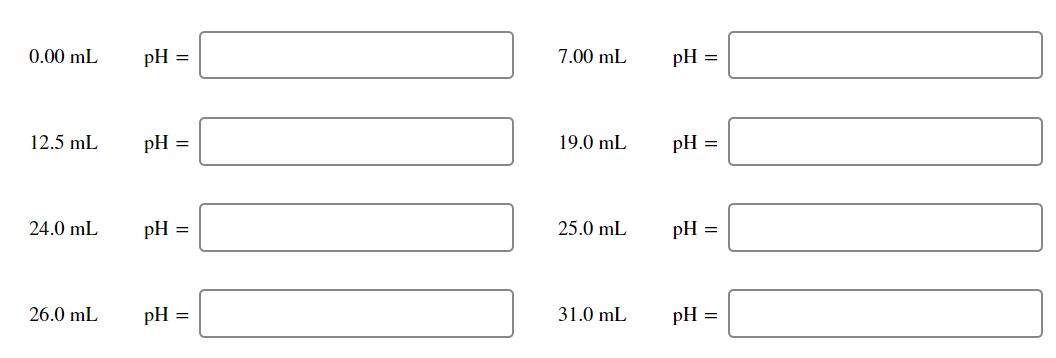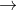In: Chemistry

# A 50.0 mL solution of 0.167 M KOH is titrated with 0.334 M HCl . Calculate...

A 50.0 mL solution of 0.167 M KOH is titrated with 0.334 M HCl . Calculate the pH of the solution after the addition of each of the given amounts of HCl .0.00 mL pH = 7.00 mL pH = 12.5 mL pH = 19.0 mL pH = 24.0 mL pH = 25.0 mL pH = 26.0 mL pH = 31.0 mL pH =

## Solutions

##### Expert Solution

Millimole of KOH in solution = Molarity x volume in mL

=0.167 M x 50 mL = 8.35 millimole

Balance equation is

KOH ( aq) + HCl ( aq)KCl ( aq) + H2O ( l )

Ionic reaction is

H+ ( aq) + OH​​​- ( aq)H2O ( l )

Nature of solution will depend on concentration of proton or hydroxyl ion and pH will be determined accordingly.

1) 0 mL

[ OH​​​​​- ] = 0.167 M

P​​​​​​OH = - log [ OH -] = - log ( 0.167 )= 0.78

P​​​​​​H = 14 -0.78 = 13.22

2) millimole of H+ = 0.334 M x 7 mL = 2.338 millimole

[ OH​​​​​-] =( 8.35 -2.338)/ ( 50+ 7 ) mL = 0.1054 M

P OH = - log ( 0.1054 ) = 0.98

P H = 14 - 0.98 = 13.02

3)12.5 mL

[ OH - ] = ( 8.35 - 4.175 ) millimole / ( 50 + 12.5 ) mL

P​​​​​H = 14 - 1.18 = 12.82

4) 19 mL

[ OH - ] = ( 8.35 - 6.35 ) millimole /( 50 + 19 ) mL

P H = 12.46

5) 24 mL

[ OH - ] = ( 8.35 -8.016 ) millimole / ( 50 + 24 ) mL

P H = 11.65

6 ) 25 mL

[ H + ​​​​​​] = [ OH - ]

P H = 7

7) 26 mL

[ H + ] = ( 8.684 - 8.35 ) millimole / ( 50+26 ) mL

P ​​​​​​H = 2.35

8 )31 mL

[ H + ] =( 10.35 - 8.35 ) millimole /( 50 + 31 ) mL

P H = 1.6

## Related Solutions

##### partb a)A 50.0-mL volume of 0.15 M HBr is titrated with 0.25 M KOH. Calculate the...
partb a)A 50.0-mL volume of 0.15 M HBr is titrated with 0.25 M KOH. Calculate the pH after the addition of 13.0 mL of KOH. Express your answer numerically. Part b) A 75.0-mL volume of 0.200 M NH3 (Kb=1.8×10−5) is titrated with 0.500 M HNO3. Calculate the pH after the addition of 15.0 mL of HNO3. Express your answer numerically.Part c) A 52.0-mL volume of 0.35 M CH3COOH (Ka=1.8×10−5) is titrated with 0.40 M NaOH. Calculate the pH after the...
##### A 25.0−mL solution of 0.100 M CH3COOH is titrated with a 0.200 M KOH solution. Calculate...
A 25.0−mL solution of 0.100 M CH3COOH is titrated with a 0.200 M KOH solution. Calculate the pH after the following additions of the KOH solution (a) 10.0 mL (b) 12.5 mL (c) 15.0 mL
##### A 26.0−mL solution of 0.120 M CH3COOH is titrated with a 0.220 M KOH solution. Calculate...
A 26.0−mL solution of 0.120 M CH3COOH is titrated with a 0.220 M KOH solution. Calculate the pH after the following additions of the KOH solution: (a) 0.00 mL (b) 5.00 mL
##### A 10.0−mL solution of 0.570 M NH3 is titrated with a 0.190 M HCl solution. Calculate...
A 10.0−mL solution of 0.570 M NH3 is titrated with a 0.190 M HCl solution. Calculate the pH after the following additions of the HCl solution a)0mL b)10ml c)30mL d)40mL
##### A 20.0-mL sample of 0.150 M KOH is titrated with 0.125 M HClO4 solution. Calculate the...
A 20.0-mL sample of 0.150 M KOH is titrated with 0.125 M HClO4 solution. Calculate the pH after the following volumes of acid have been added. 22.5 mL of the acid
##### A 83.0 mL sample of 0.0500 M HNO3 is titrated with 0.100 M KOH solution. Calculate...
A 83.0 mL sample of 0.0500 M HNO3 is titrated with 0.100 M KOH solution. Calculate the pH after the following volumes of base have been added. (a) 11.2 mL pH = _________ (b) 39.8 mL pH = __________    (c) 41.5 mL pH = ___________ (d) 41.9 mL pH = ____________    (e) 79.3 mL pH = _____________
##### A 25.0 mL solution of 0.100 M CH3COOH is titrated with a 0.200 M KOH solution....
A 25.0 mL solution of 0.100 M CH3COOH is titrated with a 0.200 M KOH solution. Calculate the pH after the following additions of the KOH solution: (a) 0.0 mL, (b) 5.0 mL, (c) 10.0 mL, (d) 12.5 mL, (e) 15.0 mL. (25 points)
##### A 25.0 mL solution of 0.100 M CH3COOH is titrated with a 0.200 M KOH solution....
A 25.0 mL solution of 0.100 M CH3COOH is titrated with a 0.200 M KOH solution. Calculate the pH after the following additions of the KOH solution: (a) 0.0 mL, (b) 5.0 mL, (c) 10.0 mL, (d) 12.5 mL, (e) 15.0 mL. (25 points)
##### 50.0 mL of a 0.100 -M HoAc solution is titrated with a 0.100 -M NaOH solution....
50.0 mL of a 0.100 -M HoAc solution is titrated with a 0.100 -M NaOH solution. Calculate the pH at each of the following points. Volume of NaOH added: 0, 5, 10, 25, 40 ,45 , 50 , 55 , 60 , 70 , 80 , 90 , 100
##### A 10.0 mL solution of 0.300 M NH3 is titrated with a 0.100 M HCl solution....
A 10.0 mL solution of 0.300 M NH3 is titrated with a 0.100 M HCl solution. Calculate the pH after the addition of 20.0 mL of the HCl solution. (potentially useful info: Ka of NH4+ = 5.6 x 10−10)